Courses

# Doc: Periodicity of Atomic Properties Class 11 Notes | EduRev

## JEE : Doc: Periodicity of Atomic Properties Class 11 Notes | EduRev

The document Doc: Periodicity of Atomic Properties Class 11 Notes | EduRev is a part of the JEE Course Chemistry for JEE.
All you need of JEE at this link: JEE

EFFECTIVE NUCLEAR CHARGE:

Between the outer most valence electrons and the nucleus of an atom, there exists finite number of shells containing electrons. Due to the presence of these intervening electrons, the valence electrons are unable to experience the attractive pull of the actual number of protons in the nucleus. These intervening electrons act as shield between the valence electrons and protons in the nucleus. Thus, the presence of intervening (shielding) electrons reduces the electrostatic attraction between the protons in the nucleus and the valence electrons because intervening electrons repel the valence electrons. The concept of effective nuclear charge allows us to account for the effects of shielding on periodic properties.

The effective nuclear charge (Zeff) is the charge felt by the valence electron. Zeff is given by Zeff = Z – s. Where Z is the actual nuclear charge (atomic number of the element) and s is the shielding (screening) constant.

Probability of finding the electron is never zero even at large distance from the nucleus. Based on probability concept, an atom does not have well-defined boundary. Hence, exact value of the atomic radius can't be evaluated. Atomic radius is taken as the effective size which is the distance of the closet approach of one atom to another atom in a given bonding state.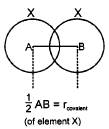It is one-half of the distance between the centres of two nuclei (of like atoms) bonded by a single covalent bond.

• Covalent radius is generally used for non-metals.

Single Bond Covalent Radius, SBCR -

(a) For Homoatomic moleucles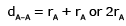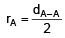• (b) For hetrodiatomic molecules in which electro negativity remains approximately same.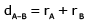• For heteronuclear diatomic molecule, A – B, where difference between the electronegativity values of atom A and atom B is relativity larger,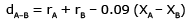where XA and Xelectronegativity values of high electronegative element A and less electronegative element B, respectively. This formula is given by Stevenson & Schomaker.

Ex.2 Calculate the bond length of C – X bond, if C – C bond length is 1.54 Å, X – X bond length is 1.00 Å and electronegativity values of C and X are 2.0 and 3.0 respectively .

Sol. (1)
C – C bond length = 1.54 Å
rC = 154/100 =  0.77 Å

rX = 100/2 = 0.50 Å

(2)  C – X bond length

dC–X = rC + r– 0.09 (Xx – XC)
= 0.77 + 0.50 – 0.09 (3 – 2)

= 0.77 + 0.50 – 0.09 × 1

= 1.27 – 0.09 = 1.18 Å

Thus C – X bond length is 1.18 Å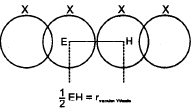It is one - half of the internuclear distance between two adjacent atoms in two nearest neighbouring molecules of the substance in solid state.

van der Waal's radius does not apply to metal. its magnitude depends upon the packing of the atoms when the element is in the solid state.

(i) The van der Waal's force of attractions are weak, therefore, their internuclear distances in case of atoms held by van der Waal's forces are much larger than those of between covalently bonded atoms.Therefore van der Waal's radii are always larger than covalent radii.

(ii) A covalent bond is formed by the overlaping of two half-filled atomic orbitals, a part of the orbital becomes common. Therefore, covalent radii are always smaller than the van der Waals radii. For example,

 Element* H O F S Br Covalent radius (A) 0.37 0.66 0.64 1.04 1.11 vander Waal's radius (A) 1,20 1.40 1.35 1.85 1.95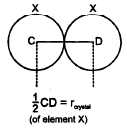It is one -half of the distance between the nuclei of two adjacent metal atoms in the metallic crystal lattice. Metallic radius of an element is always greater than its covalent radius. It is due to the fact that metallic bond (electrical attraction between positive charge of an atom and mobile electrons) is weaker than covalent bond and hence the hence the internuclear distance between the two adjacent atoms in a metallic crystal is longer than the internuclear distance between the covalently bonded atom.

For example :

 Metallic radius Covalent  radius K 231 pm 203 pm Na 186 pm 154 pm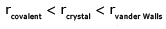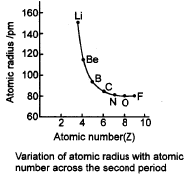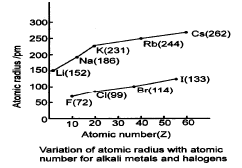Variation In a Period Variation In a Group In a period left to right: In a group top to bottom : Z increases by one unit Z increases by more than one unit Zeff. also increases Zeff. almost remains constant (due to increased screening effect of inner shells electrons) n remains constant (no of orbits) n increases (no. of orbits) As a result of these electrons are pulled close to the nucleus by the increased Zeff.rn ∝ 1/Z* Thus atomic radii decreases with increase in atomic number in a period from left to right The effect of increased number of atomic shells overweigh the effect of increased screening effect.As a result of this the size of atom increases from top to bottom in a given group.

• The atomic radius of inert gas (zero group) is given largest in a period because it is represented as van der Waals's radius which is generally larger than the covalent radius. The van der Waal's radius of inert gases also increases from top to bottom in a group.
• In the transition series (e.g. in first transition series), the covalent radii of the elements decrease from left to right across a row until near the end when the size increases slightly. On moving from left to right, extra protons are placed in the nucleus and the extra electron are added. The orbital electrons shield the nucleus charge incompletely. Thus the nuclear charge attracts all the electrons more strongly, hence a contraction in size occurs. The radii of the elements from Cr to Cu, are very close to one another because the successive addition of d-electrons screen the outer electrons (4s) from the inward pull of the nucleus. As a result of this, the size of the atom does not change much in moving from Cr to Cu.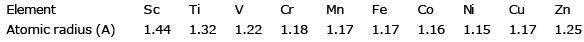• The lanthanide contraction counter balances almost exactly the normal size increase on descending a group of transition elements. Thus covalent and ionic radii of Nb (5th peroid) and Ta (6th period) are almost same due to poor shielding of f-orbitals electrons.

The effective distance from the centre of nucleus of the ion up to which it has an influence in the ionic bond is called ionic radius.

 Cation Anion It is formed by the toss of one or more electrons from the valence shell of an atom of an element.Cations are smaller than the parent atoms because(1) The whole of the outer shell of electrons is usually removed.(ii) In a Cation, the number It is formed by the gain of one or more electrons In the valence shell of an atom of an element.Anions are larger than the parent atoms because (1) Anion Is formed by gain of one or more electrons in the neutral atom and thus number of electron

For example :

 Na Na+ Number of Protons 11 11 Electronic Configuration 1s2 2s2 2p6 3s1 1s22s2 2p6 Cl Cl– Number of Electrons 17 18 Number of Proton 17 17

• The sizes of ions increases as we go down a group (cosidering the ions of same charge)
For example
Li< Na+ < K+ < Rb+ Be2+ < Mg2+ < Ca2+ < Sr2+ F < Cl < Br < I–
• The d and f orbitals do not shield the nuclear charge very effectively. Therefore there is significant reduction in the size of the ions, just after d or f orbitals have been filled completely. This is called a lanthanide contraction. Atomic radii of Zr and Hf are almost identical due to lanthanide contraction.
• The species containing the same number of electrons but differ in the magnitude of their nuclear charges are called as isoelectronic species. For example, N3- , O2-, F-, Ne, Na+ , Mg2+ and Al3+ are all isoelectronic species with same number of electrons (i.e, 10) but different nuclear charges of +7, +8, +9, +10, +11, +12 and +13 respectively.

Within a series of isoelectronic species as the nuclear charge increases, the force of attraction by the nucleus on the electrons also increases. As a result, the ionic radii of isoelectronic species decrease with increases in the magnitude of nuclear charges.

For example,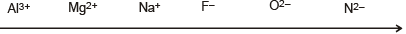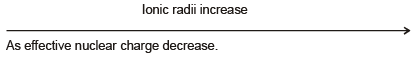• Pauling's empirical formula : Ionic radius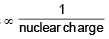• Following are the examples of isoelectronic series  (i) S2- Cl- KCa+2 Sc+3   (ii) SO2,  NO3, CO32-   (iii) N2, CO,  CN–      (iv) NH3, H3O

IONISATION ENTHALPY

lonisation enthalpy/energy (IE) , sometimes also called ionisation potential (IP) , of an element is defined as the amount of energy required to remove an electron from an isolated gaseous atom of that element resulting in the formation of positive ion.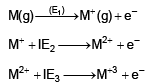IE2 & IE3 are the IInd & IIlrd ionization energies to remove electron from monovalent and divalent cations respectively.

In general: (IE)1 < (IE)2 < (IE)3 < .............
because, as the number of electrons decreases, the attraction between the nucleus'and the remaining electrons increases considerably and hence subsequent 1.E.(s) increase.

• Units of ionisation energy: KJ mol-1 ,K Cal mol-1, eV (electron volt)
• Factors Influencing lonisation enthalpy (IE) variation in a period and group may or may not be regular and can be influenced by:

(A) Size of the Atom : lonisation energy decreases with increase in atomic size. As the distance between the outermost electrons and the nucleus increases, the force of attraction between the valence shell electrons and the nucleus decreases. As a result, outer most electrons are held less firmly and lesser amount of energy is required to knock them out.

For example, ionisation energy decreases in a group from top to bottom with increase in atomic size.

(B) Nuclear Charge: The ionisation energy increases with increase in the nuclear charge. This is due to the fact that with increase in the nuclear charge, the electrons of the outer most shell are more firmly held by the nucleus and thus greater amount of energy is required to pull out an electron from the atom. For example, ionisation energy increases as we move from left to right along a period due to increase in nuclear charge.

(C) Shielding effect: The electrons in the inner shells act as a screen or shield between the nucleus and the electrons in the outermost shell. This is called shielding effect. The larger the number of electrons in the inner shells, greater is the screening effect and smaller the force of attraction and thus (IE) decreases.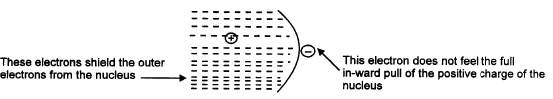(D) Penetration Effect of the Electron : The ionisation energy increases as the penentration effect of the electrons increases.

It is a well known fact that the electrons of the s-orbital has the maximum probability of being found near the nucleus and this probability goes on decreasing in case of p, d and f  orbitals of the same energy level. Within the same energy level, the penetration effect decreases in the order
s > p > d > f

Greater the penetration effect of electron more firmly the electron will be held by the nucleus and thus higher will be the ionisation energy of the atom.

For example, ionisation energy of aluminium is comparatively less than magnesium as outer most electron is to be removed from p-orbital (having less penetration effect) in aluminium where as in magnesium it will be removed from s-orbital (having large penetration effect) of same energy level.

(E) Electronic Configuration : If an atom has exactly half-filled or completely filled orbitals, then such an arrangement has extrastability.

The removal of an electron from such an atom requires more energy than expected. For example,

Be IE1 > B IE1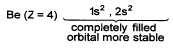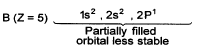As noble gases have completely filled electronic configuration, they have highest ionisation energies in their respective periods.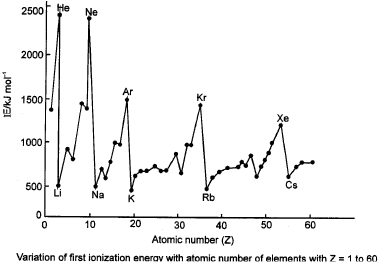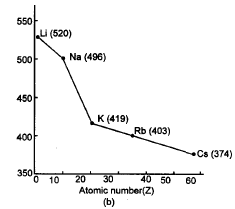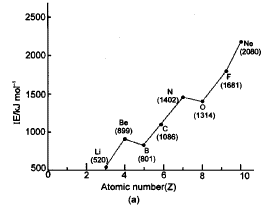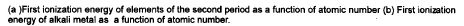• Metallic or electropositive character of elements increases as the value of ionisation energy decreases.
• The relative reactivity of the metals increases with the decrease in ionisation energy.
• The reducing power of elements increases as the value of ionisation energy decreases (Li is exception in Alkali metals group which has highest reducing power)

Ex.3 First and second ionisation energies of Mg(g) are 740 and 1450 kJ mol–1. Calculate percentage of Mg+(g) and Mg2+(g), if 1 g of Mg(g) absorbs 50 kJ of energy.

Sol. Number of moles of 1g of Mg =  1/24 = 0.0417

Energy required to convert Mg(g) to Mg+(g) = 0.0417 x 740 = 30.83 kJ
Remaining energy = 50 – 30.83 = 19.17 kJ
Number of moles of Mg2+ formed =  17.19/1450 = 0.0132

Thus, remaining Mg+ will be = 0.0417 –  0.0132 = 0.0285

% Mg+ = (0.0285/ 0.0417) × 100 = 68.35%

% Mg+ = 100 – 68.35 = 31.65%

ELECTRON GAIN ENTHALPY (ELECTRON AFFINITY) :

When an electron is added to a neutral gaseous atom (X) to convert it into a negative ion, the enthalpy change accompanying the process is defined as the electron gain enthalpy.

Electron gain enthalpy provides a measure of the ease with which an atom adds an electron to form anion.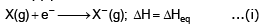Depending on the elements, the process of adding an electron to the atom can be either endothermic or exothermic. When an electron is added to the atom and the energy is released, the electron gain enthalpy is negative and when energy is needed to add an electron to the atom, the electron gain enthalpy is positive. The addition of second electron to an anion is opposed by electrostatic repulsion and hence the energy has to be supplied for the addition of second electron.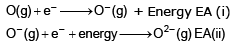EA (i) is exothermic whereas EA(ii) is endothermic.

• Group 17 elements (halogens) have very high negative electron gain enthalpies because they can attain stable noble gas electronic configuration by picking up an electron.
• Noble gases have large positive electron gain enthalpies because the electron has to enter the next higher energy level leading to a very unstable electronic configuration.
• Electron gain enthalpy of O or F is less than S or CI. This is due to the fact that when an electron is added to O or F, the added electron goes to the smaller n = 2 energy level and experiences significant repulsion from the other electrons present in this level. In S or CI, the electron goes to the larger n = 3 energy level and consequently occupies a larger region of space leading to much less electron electron repulsion.
• Electron gain enthalpies of alkaline earth metals are very less or positive because the extra electron is to be added to completely filled s-orbitals in their valence shells.
• Across a period, with increase in atomic number, electron gain enthalpy becomes more negative because left to right across a period effective nuclear charge increases and consequently it will be easier to add an electron to a small atom.
• As we move in a group from top to bottom, electron gain enthalpy becomes less negative because the size of the atom increases and the added electron would be at larger distance from the nucleus.

(i) Electron affinity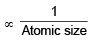(ii) Electron affinity ∞ Effective nuclear charge (zeff)

(iii) Electron affinity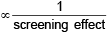(iv) Stability of half filled and completely filled orbitals of a subshell is comparatively more and the addition of an extra electron to such an system is difficult and hence the electron affinity value decreases.

Ex.4 How many CI atoms can you ionise in the process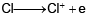he energy liberated for the process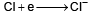or one Avogadro number of atoms.

Given IP = 13.0 eVand EA= 3.60 eV

Sol. Let n atoms be ionised. 6.02 × 1023 × EA = n × IP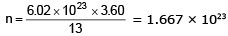Ex.5 The first ionisation potential of Li is 5.4 eV and the electron affinity of CI is 3.6 eV Calculate Δ H in kcal mol–1 for the reaction.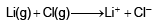Sol. The overall reaction is written into two partial equations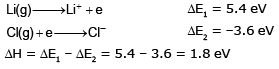= 1.8 × 23.06 kcal mol–1

= 41.508 kcal mol–1

Ex.6 For the gaseous reaction,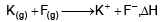was calculated to be 19 kcal under conditions where the cations and anions were prevented by electrosatic separation from combining with each other. The ionisation potential of K is 4.3 eV. What is the electron effinity of F ?

Sol.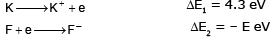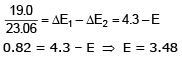Ex.7 The electron affinity of chlorine is 3.7 eV. How much energy in kcal is released when 2 g of chlorine is completely converted to CI– ion in a gaseous state? (1 eV = 23.06 kcal mol-1)

Sol.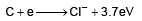35.5 3.7 × 23.06 kcal

l .'. Energy released for conversion of 2 g gaseous chlorine into CI ions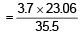× 2 = 4.8 kcal

ELECTRO-NEGATIVITY

Electronegativity is a measure of the tendency of an element to attract electrons towards itself in a covalently bonded molecules .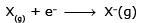The magnitude of electronegativity of an element depends upon its ionisation potential & electron affinity. Higher ionisation potential & electron affinity values indicate higher electronegativity value.

• With increase in atomic size the distance between nucleus and valence shell electrons incerases, therefore, the force of attraction between the nucleus and the valence shell electrons decreases and hence the electronegativity values also decrease.
• With increase in nuclear charge force of attraction between nucleus and the valence shell electrons increases and, therefore, electronegativity value increases In higher oxidation state, the element has higher magnitude of positive charge. Thus, due to more positive charge on element, it has higher polarising power. Thus, with increase in the oxidation state of element, its electronegativity also increases.
• Charge on cation a electronegativity of the atom
•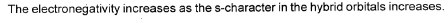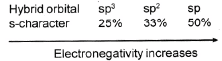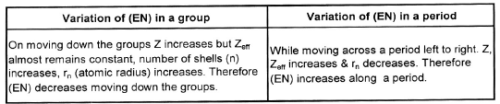There is no direct method to measure the value of electronegativity, however, there are some scales to measure its value .

(a) Pauling's Scale: Linus Pauling developed a method for calculating relative electronegativities of most elements. According to Pauling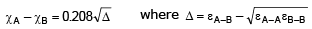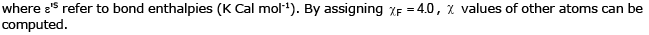(b) Mulliken's scale

Electronegativity (EN) can be regarded as the average ofthe ionisation energy (IE) and the electron affinity (EA) of an atom.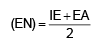If both (EA) and (IE) are determined in eV units then paulings's electronegativity (EN)p is related to Mulliken's electronegativity. Mulliken's values were about 2.8 times larger than the Pauling's values.

(c) Allred-Rochow's Electronegatlvlty Allred and Rochow defined electronegativity as the force exerted by the nucleus of an atom on its valence electrons: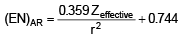where Zelleclive is the effective nuclear charge and r the covalent radius (in Å ).

• The electron negativity of Cs (55) is less than Fr (87). This is due to the increase of + 32 units in nuclear charge of Fr which makes the effective nuclear charge comparatively high.
• The electroneativity of inert gas elements of zero group is zero. Inert gases exist as monoatomic molecules and the electronegativity is the property of bonded atoms.

Ex.8 lonisation potential and electron affinity of fluorine are 17.42 and 3.45 eV respectively. Calculate the electronegativity of fluorine.

Sol. According to Mulliken equation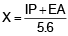when both IP and EA are taken in eV..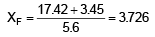APPLICATIONS OF ELECTRONEGATIVITY :

(I) Nomenclature: Compounds formed from two nonmetals are called binary compounds. Name of more electronegative element is written at the end and 'ide' is suffixed to it. The name of less electronegative element is written before the name of more electronegative element of the formula.

Ex.9 Write the correct formula and name of the following (a) ICI or CIl (b) FCI or CIF (c) BrCI or CIBr (d) BrI or IBr (e)OF2 or F2O (f)Cl2O or OCI2

Sol. Correct formula Name
(a) I+ Cl– Iodine chloride
(b) CI+ F– Chlorine fluoride
(c) Br+ CI– Bromine chloride
(d) IBr Iodine bromide
(e) OFOxygen difluoride
(f) Cl2O Dichlorine oxide

(II) Nature of Bond: If difference of electronegativities of the two elements is 1.7 or more, then ionic bond is formed between them whereas if it is less than 1.7, then covalent bond is formed. (HF is exception in which bond is covalent although difference of electronegativity is 1.9)

(iii) Metallic and Nonmetallic Nature : Generally values of electronegativity of metallic elements are low, whereas electronegativity values of nonnmetals are high.

(iv) Partial Ionic

Character in Covalent bonds Partial ionic characters are generated in covalent compounds by the difference of electronegativities.

Hanny and smith calculated percentage of ionic character from the difference of electronegativity.

Percentage of ionic character =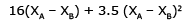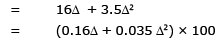XA is electronegativity of element A

XB is electronegativity of element B

Δ = XA – XB

(v) Bond length

When difference of electronegativities of atoms present in a molecule is increased, then bond length decreases. Shoemaker and stephensen determined.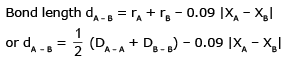(vi) Bond Strength & Stability

Bond strength and stability of A – B increases on increase in difference of electronegativities of atoms A and B bonded A – B. Therefore H – F > H – Cl > H – Br > H – I

Ex.10 Electronegativity of which of the following is high ?

(1) –CH3(sp3)
(2) H2C = CH2(sp2)
(3) CH ≡ CH(sp)
(4) Equal in all

Ans. (3)

Ex.11 CF3NH2 is not a base, whereas CH3NHis a base. What is the reason ?

Sol. Due to high electronegativity of F tendency of donating the lone pair of electrons present on N will be less

Ex.12 OF2 is called oxygen difluoride, whereas Cl2O is called dichlorine monoxide. Why ?

Sol. Electronegativity of O in OF2 is less than F. Therefore, there will be positive charge on oxygen and negative charge on fluorine. Whereas in Cl and O, electronegativity of Cl is less than that of O therefore there will be positive charge on Cl and negative charge on O. Positive charge is written first followed by negative charge.

Ex.13 Calculate the electronegativity of fluorine from the following data :

EH – H = 104.2 kcal mol–1,
EF–F = 36.6 kcal mol–1
EH–F = 134.6 kcal mol–1,
X= 2.1

Sol. Let the electronegativity of fluorine be XF.

Applying Pauling's equation.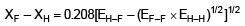In this equation, dissociation energies are taken in kcal mol–1.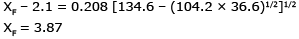Ex.14 The electron affinity of chlorine is 3.7 eV. How much energy in kcal is released when 2 g of chlorine is completely converted to Cl– ion in a gaseous state ? (1 eV = 23.06 kcal mol–1)

Sol.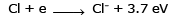35.5 3.7 × 23.06 kcal

∴ Energy released for conversion of 2 g gaseous chlorine into Cl– ions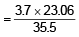× 2 = 4.8 kcal

Ex.15 Calculate the electronegativity of fluorine from following data :
EH–H = 104.2 kcal mol–1
EF–F = 36.6 kcal mol–1
EH–F = 134.6 kcal mol–1
Electronegativity of H is 2.05.
Sol.
On Paulling scale :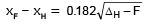(using B.E. in kcal mol–1)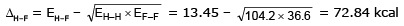From (i)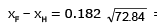= = 1.5534

xF = x+ 1.4434 = 2.05 + 1.5534 = 3.6034

(VII) METALLIC PROPERTY

Metals have the tendency to form cations by loss of electrons and this property makes the elements as electropositive elements or metals.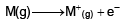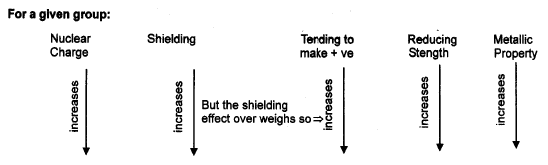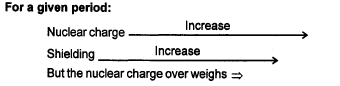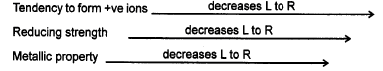(IX) OXIDES

Oxygen react with all elements except noble gases, Au, Pd and Pt to form oxides. In general, metallic oxides (O2–), peroxides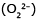and super oxides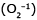are ionic solids.

The tendency of group IA metals (alkali metals) to form oxygen rich compounds increases from top to bottom i.e. with increasing cation radii and decreasing charge density on the metal ion.

IIA metals also show the similar trend. Except Be, the IIA metals react with oxygen at normal conditions to form normal ionic oxides and at high pressure of O2, they form peroxides (CaO2, SrO2, BaO2). Oxides of metals are called as basic anhydries as most of them combine with water forming hydroxides with no change in oxidation state of metals.

Oxides of IA and IIA dissolve in water forming basic solution where as other oxides do not dissolve in water.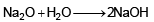Oxygen combines with many non-metals to form covalent oxides such as CO, CO2, SO2, P4O10, Cl2O7 etc.

Non-metals with limited supply of oxygen usually form oxides in which non-metals are present in lower oxidation states where as with excess of oxygen, oxides with higher oxidatin state are formed. Oxides of non-metals are called as acid anhydrides as most of them dissolve in water forming acids of oxy-acids.

P4O10 + 6H2O → 4H3 PO4 ;
SO3 + H2O → H2SO4 : Cl2O7 + H2O → 2HClO4

• In a group, basic nature of oxides increases or acidic nature decreases. Oxides of the metals are generally basic and oxides of the non-metals are acidic. The oxides of the matalloids are amphotetric in nature. The oxides of Al, Zn, Sn, As and Sb are amphoteric.
• In a period the nature of the oxides varies from basic to acidic.
 Na2O MgO Al2O3 SiO2 P4O10 SO3 Cl2O7 StronglyAcidic basic Basic amphoteric Weakly Acidic acidic Acidic

• Strongly acidic CO, N2O, NO and H2O are neutral oxides.
Offer running on EduRev: Apply code STAYHOME200 to get INR 200 off on our premium plan EduRev Infinity!

## Chemistry for JEE

223 videos|452 docs|334 tests

,

,

,

,

,

,

,

,

,

,

,

,

,

,

,

,

,

,

,

,

,

;